DECLINE CURVE BASICS
Virtually all oil and gas wells produce at a declining rate over time. The initial flow rate may be held constant on purpose (restricted rate) or the decline may begin immediately. The ultimate recovery from the well (reserves) can be calculated by projecting the decline rate forward in time to an economic limit. The projected production can be summed to find the total production on decline, and this can be added to the production during the constant rate period to obtain the ultimate recovery.

This can be done on  a per well basis or for an entire reservoir. The result can be used as a control on the volumetric reserves calculated from log analysis results and geological contouring of field boundaries. It is often used to estimate the recovery factor by comparing ultimate recovery with original oil in place or gas in place calculations.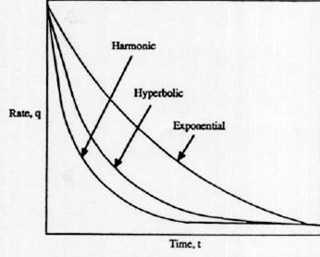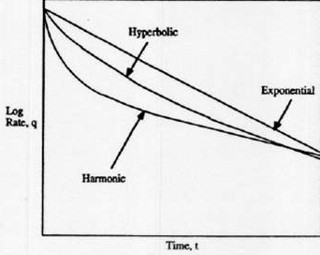Production history plot: linear (cartesian coordinate (left), semi-logarithmic plot (right). Most oil wells and some gas wells produce with an exponential decline (straight line on logarithmic plot). Some oil and gas wells decline at a faster rate, called hyperbolic or double exponential decline. Still faster declines can occur, especially in fractured reservoirs, called harmonic decline.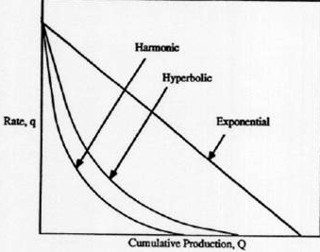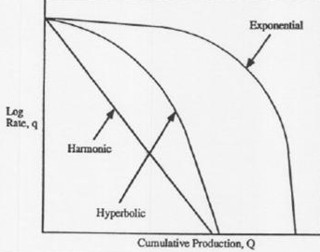Cumulative production plot: linear (cartesian coordinate (left), semi-logarithmic plot (right). Ultimate recovery is highest for exponential decline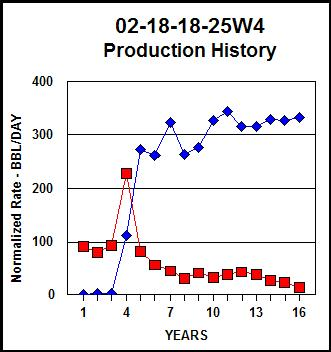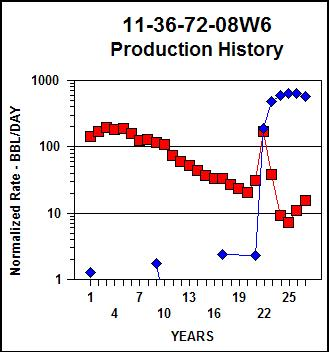Production history graphs for two real wells: linear scale (left), semi-log scale (right). Both show
restricted initial rate followed by exponential decline (red = oil, blue = water production).Determining decline rate and Ultimate recovery```
Natural decline trend is dictated by the reservoir drive mechanism, rock and fluid properties, well completion, and production practices. Thus, a major advantage of this decline trend analysis is inclusion of all production and operating conditions that would influence the performance of the well. Conversely, predictions from the decline history assumes that no significant changes in these factors will take place.

The generalized decline curve is described by::
1: D - Di * (Q / Qi)^N

Where:
D = instantaneous decline rate at time T
Di = initial decline rate
Q = instantaneous flow rate
Qi =initial flow rate at start of decline
N= 0 for exponential decline
= 1 for harmonic decline
= 2 for hyperbolic decline

Production as a function of time on decline:
Exponential decline
2: Q = Qi * exp (-Di * T)

Harmonic decline
3: Q = Qi / (1 + Di * T)

Hyperbolic decline
4: Q = Qi / (1 + N * Di * T)

NOTE: Q, D, and T must be in compatible units (egL bbl/day, 1/day, and days respectively, or month or year)

The cumulative production for each case is:
Exponential decline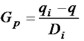5: Np = (Qi - Q) / Di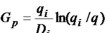Harmonic decline
6: Np = (Qi / Di) * ln (Qi / Q)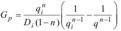Hyperbolic decline
7: Np = (Qi^N) / (Di * (1 - N)) * ((1 / (Qi^(N - 1)) - (1 / (Q^(N - 1)))

Where:
Np = cumulative production to time T

Np is usually replaced by the symbol Gp when the reservoir is a gas zone.

The time to abandonment for each case is:
Exponential decline
8: Ta = (1 / Di) * ln (Qi / Qa)

Harmonic decline
9: Ta = (1 / Di) * (Qi / Qa - 1)

Hyperbolic decline
10: Ta = (1 / (N * Di)) * ((Qi / Qa)^N - 1)

Where:
Ta = time to abandonment
Qa = production rate at abandonment

NOTE: To find ultimate recovery (reserves), calculate abandonment time (Ta), then use Ta as the time term  in equations 5, 6, or7.

Page Views ---- Since 01 Jan 2015
Copyright 2023 by Accessible Petrophysics Ltd.
CPH Logo, "CPH", "CPH Gold Member", "CPH Platinum Member", "Crain's Rules", "Meta/Log", "Computer-Ready-Math", "Petro/Fusion Scripts" are Trademarks of the Author